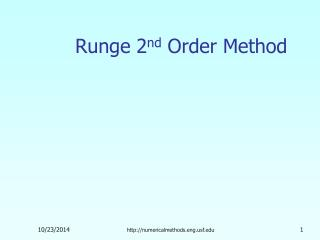DownloadDownload PresentationRunge 2 nd Order Method

# Runge 2 nd Order Method

Télécharger la présentation## Runge 2 nd Order Method

- - - - - - - - - - - - - - - - - - - - - - - - - - - E N D - - - - - - - - - - - - - - - - - - - - - - - - - - -
##### Presentation Transcript

1. Runge 2nd Order Method http://numericalmethods.eng.usf.edu

2. Runge-Kutta 2nd Order Methodhttp://numericalmethods.eng.usf.edu

3. Runge-Kutta 2nd Order Method For Runge Kutta 2nd order method is given by where http://numericalmethods.eng.usf.edu

4. y yi+1, predicted yi x xi+1 xi Heun’s Method Heun’s method Here a2=1/2 is chosen resulting in where Figure 1 Runge-Kutta 2nd order method(Heun’s method) http://numericalmethods.eng.usf.edu

5. Midpoint Method Here is chosen, giving resulting in where http://numericalmethods.eng.usf.edu

6. Ralston’s Method Here is chosen, giving resulting in where http://numericalmethods.eng.usf.edu

7. How to write Ordinary Differential Equation How does one write a first order differential equation in the form of Example is rewritten as In this case http://numericalmethods.eng.usf.edu

8. Example A ball at 1200K is allowed to cool down in air at an ambient temperature of 300K. Assuming heat is lost only due to radiation, the differential equation for the temperature of the ball is given by Find the temperature at seconds using Heun’s method. Assume a step size of seconds. http://numericalmethods.eng.usf.edu

9. Solution Step 1: http://numericalmethods.eng.usf.edu

10. Solution Cont Step 2: http://numericalmethods.eng.usf.edu

11. Solution Cont The exact solution of the ordinary differential equation is given by the solution of a non-linear equation as The solution to this nonlinear equation at t=480 seconds is http://numericalmethods.eng.usf.edu

12. Comparison with exact results Figure 2. Heun’s method results for different step sizes http://numericalmethods.eng.usf.edu

13. Effect of step size Table 1. Temperature at 480 seconds as a function of step size, h (exact) http://numericalmethods.eng.usf.edu

14. Effect of step size Table 1. Temperature at 480 seconds as a function of step size, h (exact) http://numericalmethods.eng.usf.edu

15. Effects of step size on Heun’s Method Figure 3. Effect of step size in Heun’s method http://numericalmethods.eng.usf.edu

16. Comparison of Euler and Runge-Kutta 2nd Order Methods Table 2. Comparison of Euler and the Runge-Kutta methods (exact) http://numericalmethods.eng.usf.edu

17. Comparison of Euler and Runge-Kutta 2nd Order Methods Table 2. Comparison of Euler and the Runge-Kutta methods (exact) http://numericalmethods.eng.usf.edu

18. Comparison of Euler and Runge-Kutta 2nd Order Methods Figure 4. Comparison of Euler and Runge Kutta 2nd order methods with exact results. http://numericalmethods.eng.usf.edu

19. Additional Resources For all resources on this topic such as digital audiovisual lectures, primers, textbook chapters, multiple-choice tests, worksheets in MATLAB, MATHEMATICA, MathCad and MAPLE, blogs, related physical problems, please visit http://numericalmethods.eng.usf.edu/topics/runge_kutta_2nd_method.html

20. THE END http://numericalmethods.eng.usf.edu